## 10.2 Changing the Position of a Legend

### 10.2.1 Problem

You want to move the legend from its default place on the right side.

### 10.2.2 Solution

Use `theme(legend.position = ...)`. It can be put on the top, left, right, or bottom by using one of those strings as the position (Figure 10.2, left).

``````pg_plot <- ggplot(PlantGrowth, aes(x = group, y = weight, fill = group)) +
geom_boxplot() +
scale_fill_brewer(palette = "Pastel2")

pg_plot +
theme(legend.position = "top")``````

The legend can also be placed inside the plotting area by specifying a coordinate position, as in `legend.position = c(.8, .3)` (Figure 10.2, right). The coordinate space starts at (0, 0) in the bottom left and goes to (1, 1) in the top right.

``````pg_plot +
theme(legend.position = c(.8, .3))``````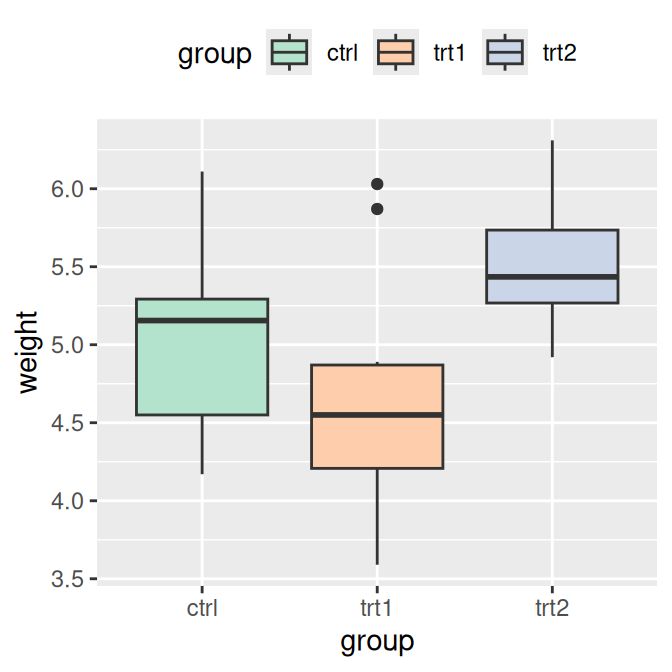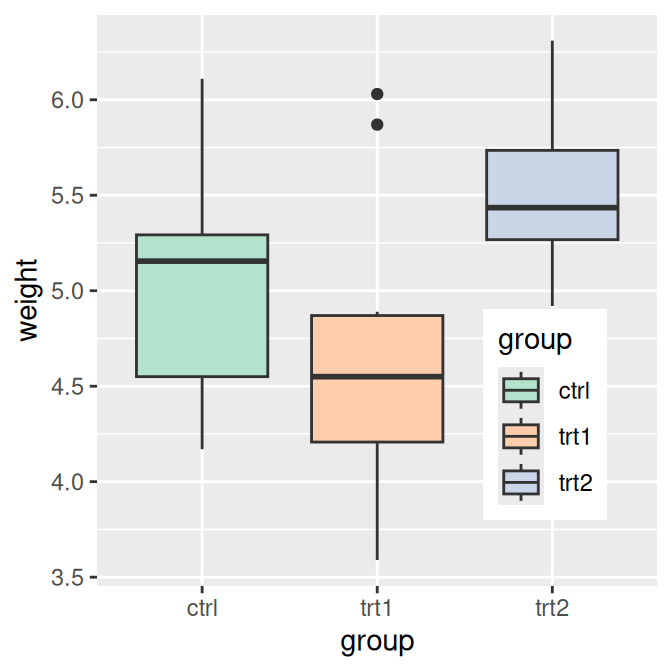Figure 10.2: Legend on top (left); Legend inside of plotting area (right)

### 10.2.3 Discussion

You can also use `legend.justification` to set which part of the legend box is set to the position at `legend.position`. By default, the center of the legend (.5, .5) is placed at the coordinate, but it is often useful to specify a different point.

For example, this will place the bottom-right corner of the legend (1, 0) in the bottom-right corner of the plotting area (1, 0) (Figure 10.3, left):

``````pg_plot +
theme(legend.position = c(1, 0), legend.justification = c(1, 0))``````

And this will place the top-right corner of the legend in the top-right corner of the graphing area (Figure 10.3, right):

``````pg_plot +
theme(legend.position = c(1, 1), legend.justification = c(1, 1))``````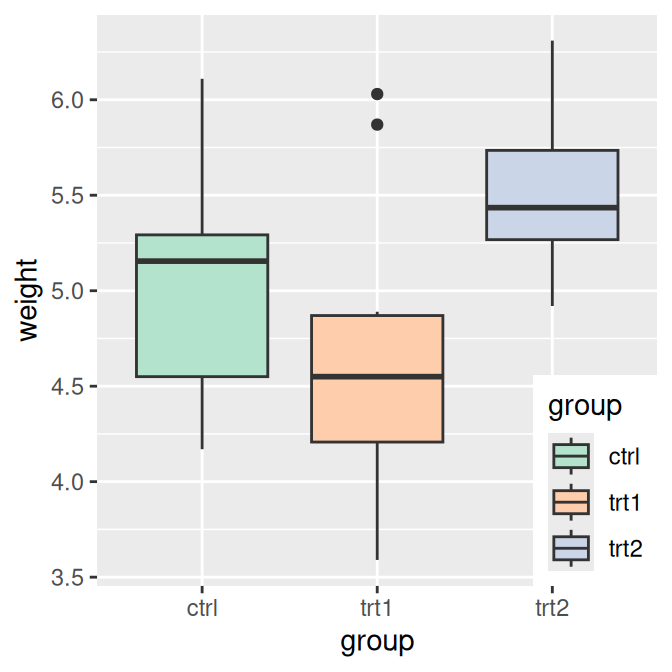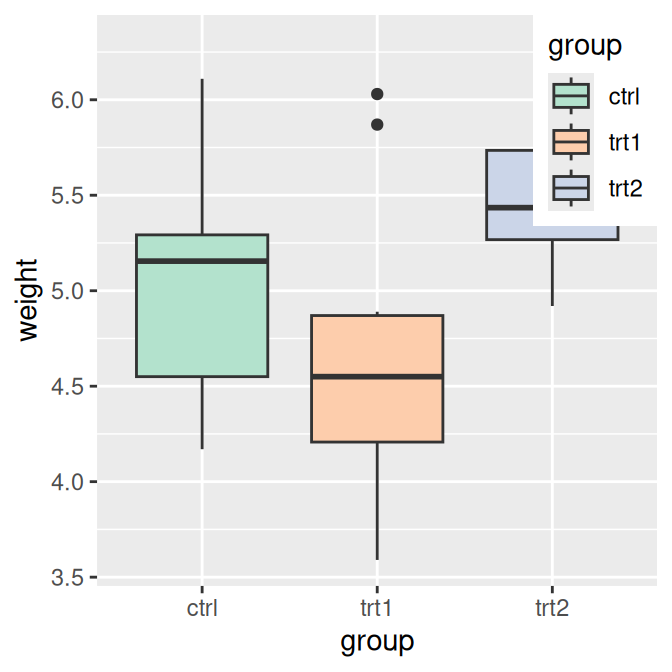Figure 10.3: Legend in bottom-right corner (left); Legend in top-right corner (right)

When placing the legend inside of the graphing area, it may be helpful to add an opaque border to set it apart (Figure 10.4, left):

``````pg_plot +
theme(legend.position = c(.85, .2)) +
theme(legend.background = element_rect(fill = "white", colour = "black"))``````

You can also remove the border around its elements so that it blends in (Figure 10.4, right):

``````pg_plot +
theme(legend.position = c(.85, .2)) +
theme(legend.background = element_blank()) +  # Remove overall border
theme(legend.key = element_blank())           # Remove border around each item``````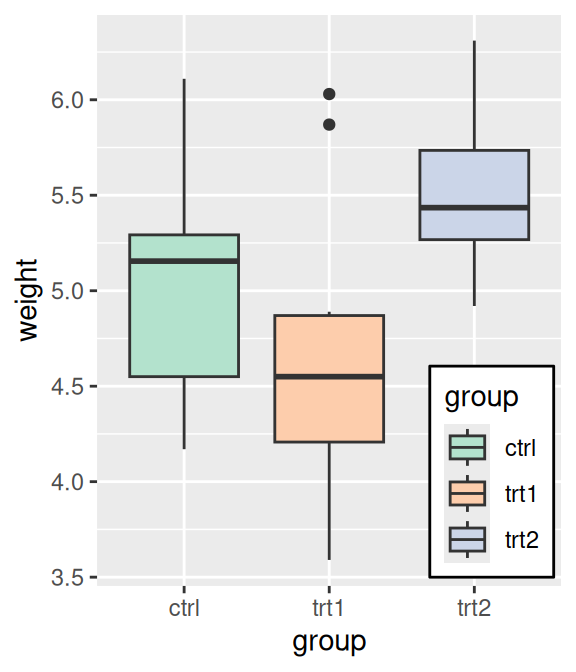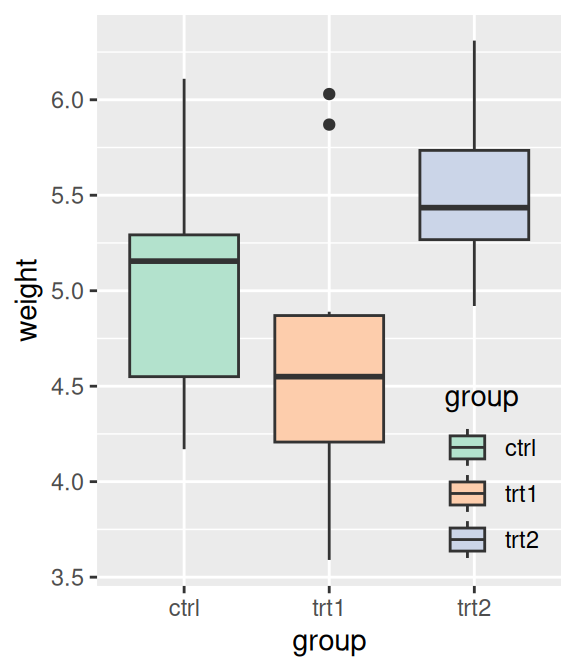Figure 10.4: Legend with opaque background and outline (left); With no background or outlines (right)